Mobile QR Code1. (Department of Electric Engineering, Gangneung-Wonju National University, Gangneung, 25457, Korea)

Pass gate effect (PGE), DRAM, BCAT, shallow trench isolation (STI)

## I. INTRODUCTION

The dynamic random-access memory (DRAM) cell consists of one access transistor and one capacitor (1T1C). Precisely, the 1T1C structure provides high performance and high reliability. Meanwhile, the present growth of artificial intelligence, high-performance computers, and big data industries requires more data to be processed in DRAM. To satisfy requirements such as high operation speed, density, and power efficiency, the DRAM has been developed via a down-scale approach. However, as the down-scaling progresses, the refresh time decreases due to an increase in leakage currents that are such a gate induced drain leakage (GIDL), gate induced junction leakage (GIJL), pass gate effect (PGE), and 1-row hammer [1-7]. Further, when the technology node of the DRAM is above 20 nm, the effects of the GIDL and GIJL, owing to band to band tunneling, under the gate region are critical. Then, when the technology node is less than 20 nm, the distance between adjacent cell devices is extremely short, which results in a significant increase in the adjacent cell interference characteristics. The interference between adjacent cells is causing PGE and 1-row hammer. Specifically, the PGE and 1-row hammer which occur because of the decreasing word line (WL to WL) distance, become more critical problems than GIDL and GIJL in the DRAM. Nevertheless, the error cell generated by GIDL and GIJL are fixed by incorporating error correcting code (ECC) [8-10]. However, since PGE and 1-row hammer cause simultaneous error bits in one word line (WL), fixing with an ECC becomes difficult. Therefore, to avoid interferences between adjacent cells, it is important to reduce the PGE in recent DRAM technology.

In this study, we propose a spherical shallow trench isolation (STI) structure that located at STI surface to reduce for PGE. The spherical STI structure was verified using TCAD. We measured PGE according to spherical STI location and radius for optimizing structure. As a result, we found that optimized location and as radius increased, the PGE was decreased. Furthermore, PGE was analyzed according to the trap density formed on the STI surface in our proposed structure. As trap density was high, the PGE was reduced. On the other hand, trap density was important parameter for PGE. So, to organize, we investigated many important parameters for PGE to optimize the structure proposed with spherical STI.

## II. DRAM STRUCTURE AND SIMULATION CONDITION

Fig. 1(a) (6F$^{2}$ DRAM) shows the layout of the DRAM in the unit array area, which is presently widely used. More precisely, the DRAM structure comprises one common bit line, two buried silicon active word lines (AWL), and a saddle fin (Fig. 1(b)). Fig. 2 shows the half-cross view of the DRAM cell we constructed a structure which the field pass-word line (FPWL) outside the cell was added to measure the PGE. In addition, dimensions are close to the typical DRAM cell technology node 1y and the corresponding simulation conditions (Table 1). Specifically, when the FPWL is in the turn-off'' state and the turn-on state, the threshold voltage (V$_{\mathrm{th}}$) value is measured, and the two V$_{\mathrm{th}}$ differences are defined by the PGE.

##### Fig. 1. (a) Diagram of 6F2 DRAM array top view and relative position of field pass gate and victim cells; (b) 3D BCAT structure cross view which used for transistor simulation.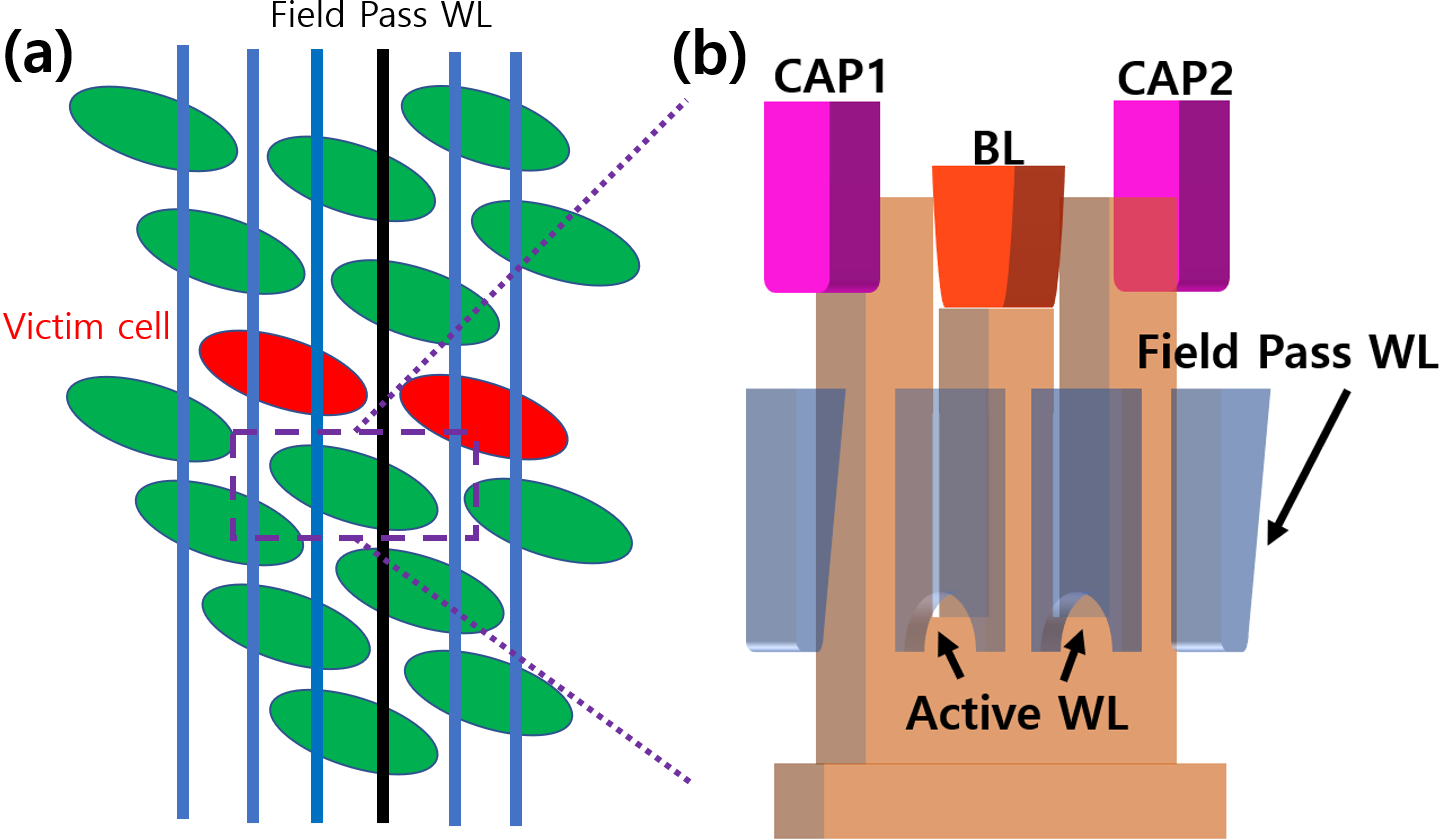##### Fig. 2. Cross half section of 2D BCAT structure.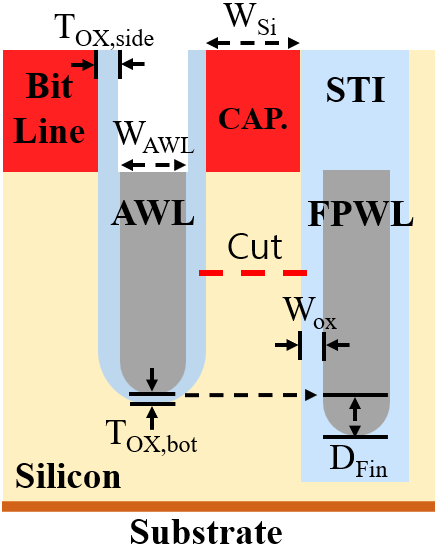##### Table 1. Structure condition and simulation condition
 Structure condition Simulation condition Region [nm] Region Voltage [V] Gate oxide thickness side/bottom (Tox_side/Tox_bot) 8/6 BL voltage 1 Silicon active width (Wsi) 23 Silicon active WL voltage (Von/Voff) 3/-0.2 Shallow trench isolation width (WSTI) 28 Field Pass WL voltage (Von/Voff) 3/-0.2 World line width (WAWL, WFPWL) 12 Substrate voltage -0.6 Fin depth (DFin) 20

## III. SIMULATION RESULTS

Fig. 3 shows the energy band of the victim cell according to the FPWL voltage. Specifically, the electric field created by the FPWL voltage affects the active channel region. As a results, energy barrier of the victim cell is lowered by the electric field, because the threshold voltage (V$_{\mathrm{th}}$) is reduced. When a leakage current occurs due to a decrease in V$_{\mathrm{th}}$, the stored data in the capacitor disappears because of the sub-threshold current. In particular, the occurrence of PGE causes data loss. It is important to prevent data loss in DRAM since it is, the main storage device.

To facilitate quantification and comparison, it is necessary to define the PGE before analyzing it. Fig. 4 demonstrates the relationship between the on/off states of the FPWL and AWL V$_{\mathrm{th}}$ in the I$_{d}$V$_{g}$ curve of the BCAT structure. In this study, we define the PGE as V$_{\mathrm{th}}$ difference of the victim cell extracted by constant current method at the point of 10$^{-7}$ current.

In this work, we altered various dimensions to find out the important influence of the DRAM structure on the PGE and consequently interpreted PGE via geometric analysis. More specifically, as the physical distance between the AWL and FPWL increased, the PGE received by the victim cell decreased. Furthermore, the thinner the gate oxide thickness bottom, the better the channel control, which reduces the interference of the pass gate voltage (Fig. 5). Additionally, as the physical distance between the AWL and the FPWL increased, the PGE received by the victim cell decreased.

From another perspective, the more the channel was affected by the AWL voltage, the less the PGE. Furthermore, the PGE according to STI width and silicon active width were measured. (Fig. 6 and 7) STI width is a more critical variable than silicon active width.

However, in general, the physical distance between the AWL and FPWL cannot be increased because the cell size of the DRAM cannot be expanded. In addition, the thickness of the AWL bottom oxide thickness is limited. Thus, we should reduce the PGE with fixed distances.

##### Fig. 3. The simulation results of 2D BCAT structure: band diagram along the red dashed cut lines in Fig. 2.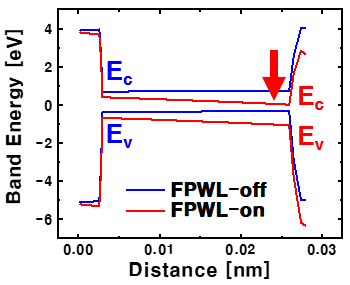##### Fig. 4. The simulation results of 2D BCAT structure: I$_{\mathrm{d}}$V$_{\mathrm{g}}$ curve. We defined the voltage difference at the point of 10$^{-7}$ current as PGE.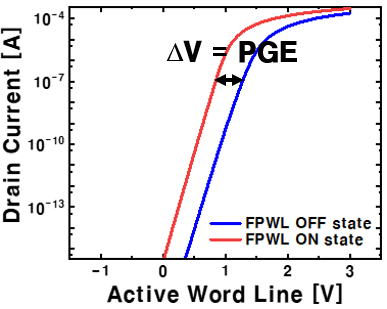##### Fig. 5. Measure PGE while modifying gate oxide thickness bottom.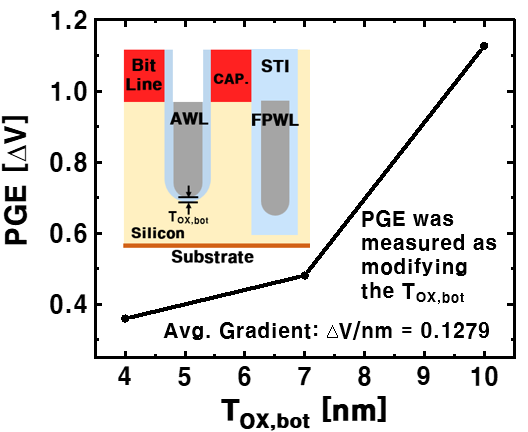##### Fig. 6. Measure PGE while modifying silicon active width.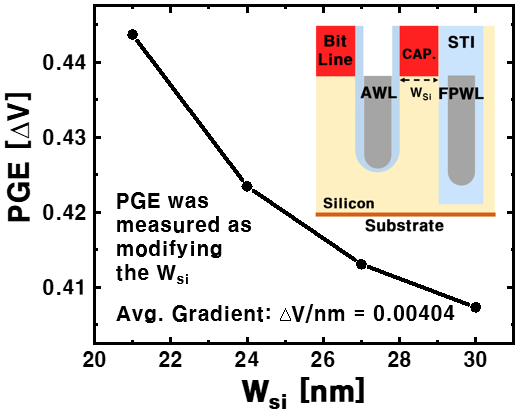##### Fig. 7. Measure PGE while modifying STI width.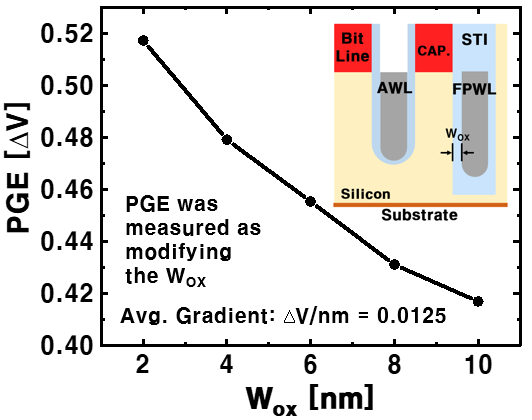## IV. OPTIMIZING THE PGE ACCORDING TO DIMENSION

We measured the PGE according to the occupied T$_{\mathrm{ox}}$,$_{\mathrm{side}}$ and W$_{\mathrm{AWL}}$ while maintaining a fixed distance between BL and drain. Fig. 8 shows the PGE according to the length of T$_{\mathrm{ox}}$,$_{\mathrm{side}}$ The thinner the T$_{\mathrm{ox}}$,$_{\mathrm{side}}$, the more fully the AWL voltage is applied to the channel region. The influence of FWPL voltage on the channel is reduced.

Therefore, when T$_{\mathrm{ox}}$,$_{\mathrm{side}}$ decreased reduces PGE.

Furthermore, we measured PGE according to the occupied W$_{\mathrm{si}}$ and W$_{\mathrm{ox}}$ while maintaining a fixed distance between AWL and FPWL. Specifically, the PGE decreases as W$_{\mathrm{si}}$ increases (Fig. 9). Further, Silicon and SiO$_{2}$ have different permittivity. Therefore, as the ratio of SiO$_{2}$ with a large dielectric constant increase, the capacitance at between AWL and FPWL increases. As a result, the effect of the FWPL voltage decreases.

We verified the tendency of PGE measured through the equivalent circuit of MOSFET. Fig. 10(a) shows the PGE relation formula derived through the equivalent circuit and equivalent circuit of MOSFET. Through the division capacitance formula, we calculated net voltage. PGE is proportional to the voltage applied between T$_{\mathrm{ox}}$ and C$_{\mathrm{si}}$. The tendency of PGE according to W$_{\mathrm{si}}$ was identified. As a results, we confirmed the same tendency of PGE according to W$_{\mathrm{si}}$ as measured through experiments (Fig. 10(b)).

##### Fig. 8. PGE was measured while varying the ratio of T$_{\mathrm{OX,side}}$ and W$_{\mathrm{AWL}}$: Fix physical distance of BCAT width.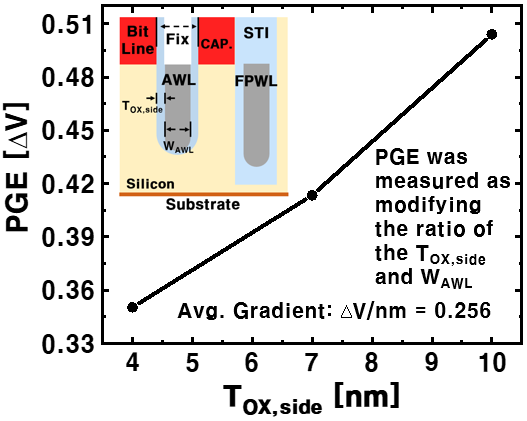##### Fig. 9. PGE was measured as varying the ratio of W$_{\mathrm{Si}}$ and W$_{\mathrm{ox}}$: Fix physical distance of active width.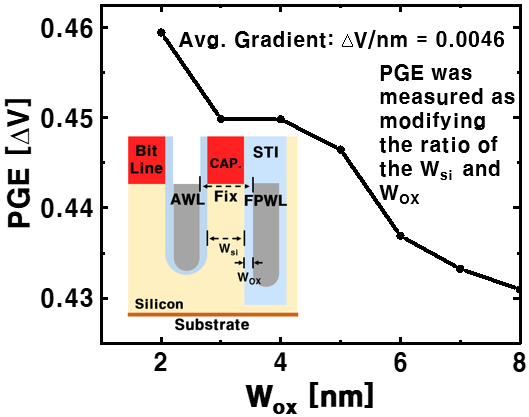##### Fig. 10. (a) A mathematical formula of total capacitance and Equivalent Circuit; (b) PGE tradeoff between W$_{\mathrm{si}}$ and W$_{\mathrm{ox}}$.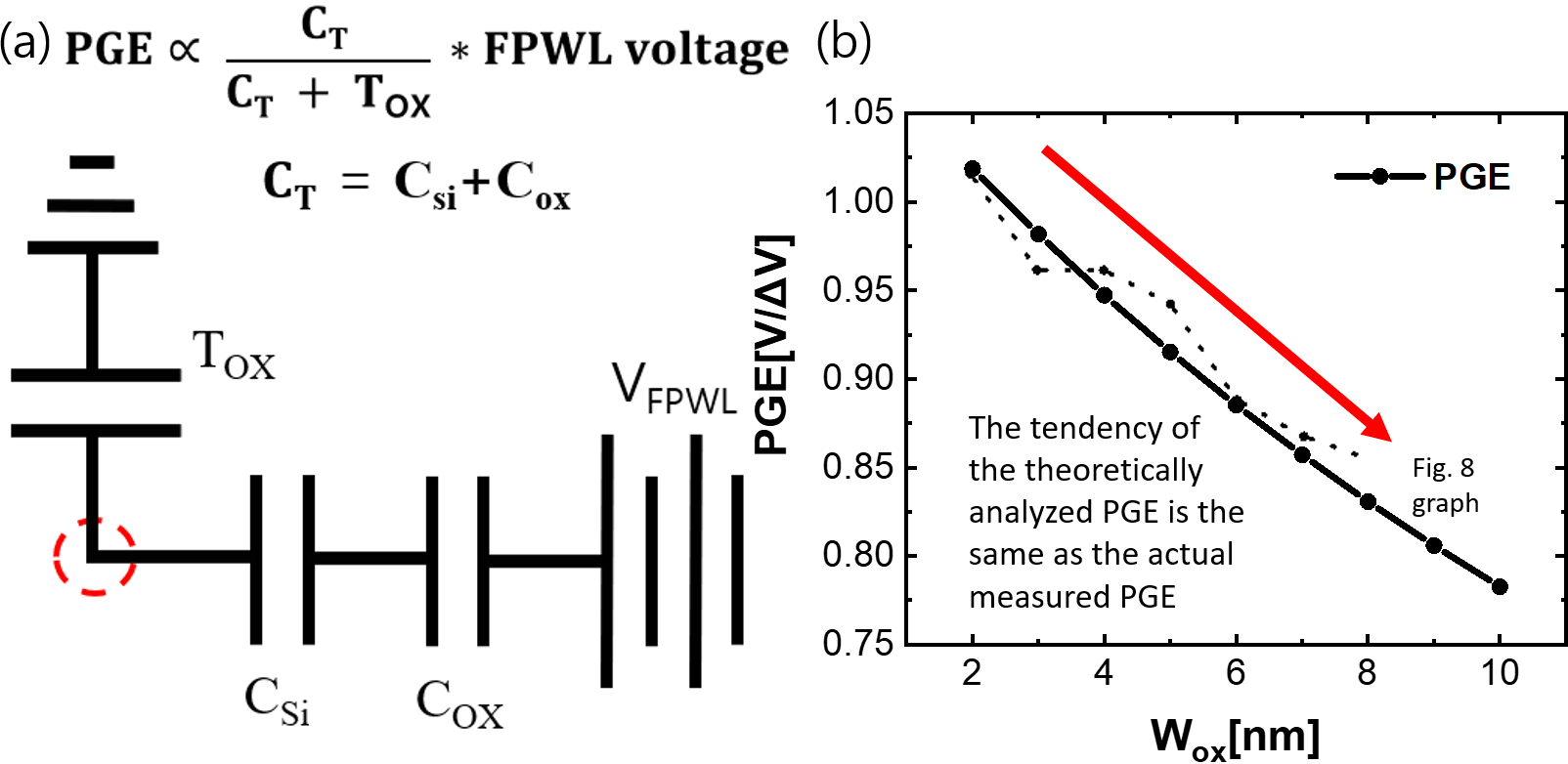## V. PROPOSE SPHERICAL STI STRUCTURE

If the ratio of SiO$_{2}$ to silicon, between AWL and FPWL is high PGE can be reduced. However, there is a limit to increasing SiO$_{2}$. Further, as the area of silicon decreases, the contact resistance of the metal line, in contact with silicon, increases. More specifically, we propose to add a physical STI structure to overcome the limitations (Fig. 11). Particularly, by adding the spherical STI structure, the metal line contact resistance is reduced, and the ratio occupied by SiO$_{2}$ is increased. Fig. 12 depicts the fabrication process used to create the spherical STI. Specifically, Fig. 12(a) and (b) demonstrate the utilization of dry etching process to generate spherical STI at a specific depth on a single crystal silicon wafer. The spherical shape is obtained through a wet etching process with isotropic characteristic. Finally, the desired depth of the STI is achieved through the dry etching process (Fig. 12(d)).

Also, we measured PGE according to the structural position and radius length to optimize the spherical STI structure. Fig. 13 shows the PGE according to the spherical STI position. The strength of the E-field created by the FWPL voltage was maximum at Depth 175 nm. As a result, the PGE was reduced to the lowest by adding the spherical STI when the Depth was 175 nm. Fig. 14 shows the PGE according to the length of the radius. The PGE was reduced in proportion to the size of the radius. This shows the same tendency as the previous experiment.

##### Fig. 11. Spherical STI 2D BCAT structure.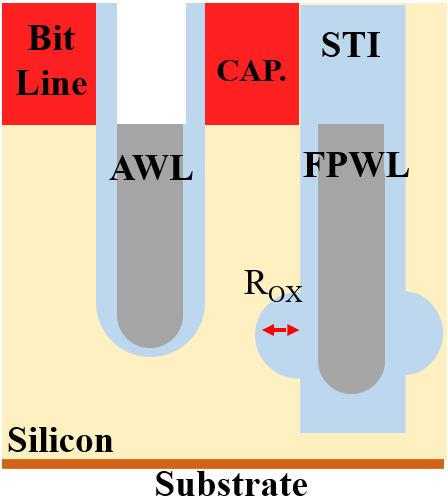##### Fig. 12. Fabrication of spherical STI on single crystal silicon wafer. We adopted a dry-wet-dry etching process while the STI process.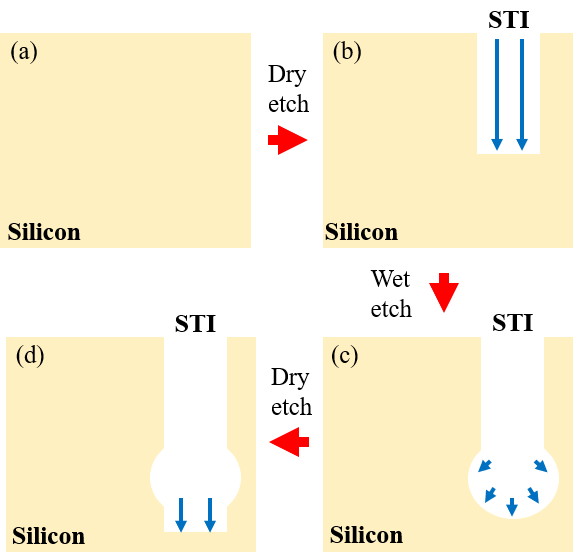##### Fig. 13. The simulation results of spherical STI 2D BCAT structure: PGE according to spherical STI location.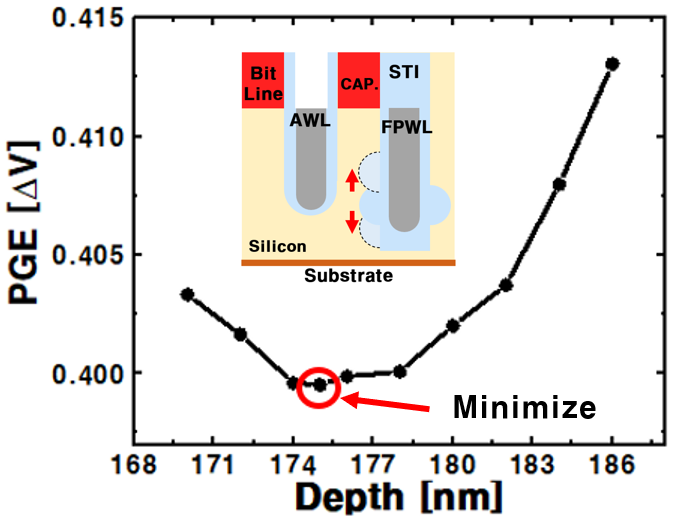##### Fig. 14. PGE according to spherical STI radius. The PGE decreased as the radius increased.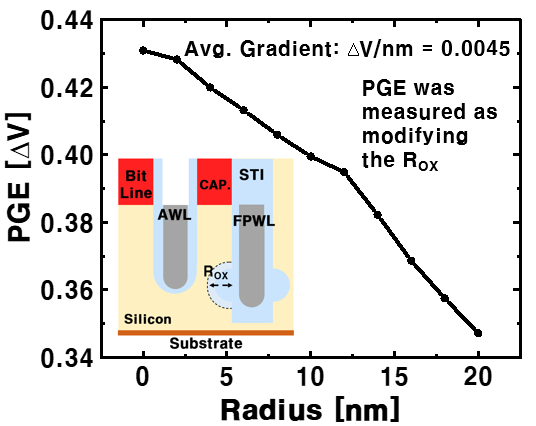## VI. PGE BY TRAP DENSITY

Furthermore, we measured the PGE according to the trap density of the surface STI, further from our proposed spherical STI structure we proposed. In particular, the V$_{\mathrm{th}}$ varies according to the trap density of the STI surface. So, we adjusted the body doping concentration to make it the same as the V$_{\mathrm{th}}$ of the previously measured structures. As a result, we fully measured PGE according to trap density. Fig. 15 shows the change in PGE according to Trap density. More precisely, as the trap density increases, the PGE decreases. The electrons captured on the STI surface serve as screens to reduce the electric field between the FPWL and the AWL. In addition, if the trap density increases above a certain level, the PGE hardly decreases. Sufficiently captured electrons can extremely reduce the voltage interference of FPWL with AWL. Therefore, the trap generated on the surface of STI is an important variable affecting PGE.

##### Fig. 15. PGE according to trap density as spherical STI radius is 10 nm. The green zone is the trap area. The PGE was measured when the trap density was above a certain level.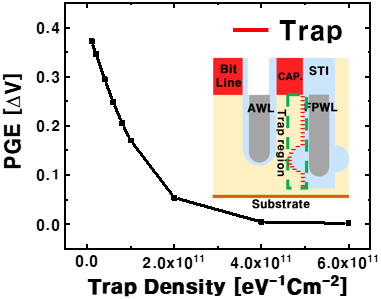## VII. CONCLUSIONS

In this study, we analyzed the pass gate effect (PGE) appearing in the dynamic random-access memory (DRAM) transistor using the energy band. More precisely, to find out the parameters that affect PGE, the PGE of tendency was measured while modifying various dimensions in the widely adopted buried channel array transistor (BCAT) structure. Based on the measured results, we proposed a spherical shallow trench isolation (STI) structure to reduce PGE while fixing the DRAM cell size. Additionally, we optimized the position of the spherical STI structure and the length of the radius. Furthermore, the trap density was optimized on the STI surface to reduce the PGE. In the TCAD simulation, the PGE is reduced by approximately 4 orders compared to the DRAM BCAT structure currently adopted.

## ACKNOWLEDGMENTS

This paper was supported by research funds for newly appointed professors of Gangneung-Wonju National University in 2021 and the National Research Foundation of Korea (NRF) grant funded by the Korea government (MSIT) (2021R1G1A1093786). This research was supported by the National R&D Program through the National Research Foundation of Korea (NRF) funded by the Ministry of Science and ICT (NRF- 2022M3I7A1078936). This research was supported by "Regional Innovation Strategy (RIS)" through the National Research Foundation of Korea (NRF) funded by the Ministry of Education (MOE)(2022RIS-005)

## References

1
Yumneg Sun, Xiang Liu, et al, “Trap-Assisted Passing Word Line Leakage and Variable Retention Time in DRAM,” 2021 IEEE 4th International Conference on Electronics Technology(ICET), IEEE Journal of, June., 2021, doi: 10.1109/ICER51757.2021.94510592
Xiaobin Yuan, Jae-Eun Park, et al, “Gate-Induced-Drain-Leakage Current in 45-nm CMOS Technology,” IEEE Transactions on Device and Materials Reliability, Vol. 8, No. 3, pp. 501-508, Sep., 2008, doi: 10.1109/TDMR.2008.20023503
K. Y. Lim, X. Yu, et al, “A study on gate-induced junction breakdown,” 2001 6th International Conference on Solid-State and Interated Circuit Technologly. Proceedings, IEEE Journal of, Aug., 2002, doi: 10.1109/ICSICT.2001.9820524
G.Q. Lo, A. B Joshi, “Hot-carrier-stress effects on gate-induced drain leakage current in n-channel MOSFETs,” IEEE Electron Device Letters, Vol. 12, No. 1, pp. 5-7, Jan., 1991, doi: 10.1109/55.756795
C. Chang, S. Haddad, et al, “Drain-avalanche and hole-trapping induced gate leakage in thin-oxide MOS device,” IEEE Electron Device Letters, Vol. 9, No. 11, pp. 588-590, Nov., 1988, doi: 10.1109/55.92856
Thomas Yang, Xi-Wei Lin, “Trap-Assisted DRAM Row Hammer Effect,” IEEE Electron Device Letters, Vol. 40, No. 3, pp. 391-394, Jan., 2019, doi: 10.1109/LED.2019.28912607
Mungyu Son, Hyunsun Park, “Making DRAM strong against row hammering,” 2017 54th ACM/EDAC/IEEE Design Automation Conference (DAC), IEEE journal of, Oct., 2017, doi: 10.1145/3061639.30622818
Yinyi Lin, J.K. Wolf, “Combined ECC/RLL code,” IEEE Transactions on Magetics, Vol. 24, No. 5, pp. 2527-2529, Nov., 1998, doi: 10.1109/20.921639
Sanghyuk Kwon, Young Hoon Son, et al, “Understanding DDR4 in pursuit of In-DRAM ECC,” 2014 International SoC Design Conference (ISOCC), IEEE Journal of, Apr., 2015, doi: 10.1109/ISOCC.2014.708764610
T. Yamada, H. Kotani, et al, “A 4-Mbit DRAM with 16-bit concurrent ECC,” IEEE Journal of Solid-State Circuits, Vol. 23, No. 1, pp. 20-26, Feb., 1988, doi: 10.1109/4.251Yeon Seok Kim has been studying in the Department of Electronic Engineering at Gangneung-Wonju National University (GWNU, Korea) from 2019 to 2023, also he has now been working on M.S. course at GWNU. His current research interests include MOS devices for DRAM memory at the Intelligent Semiconductor Device & Circuit Design Laboratory (ISDL) according to Professor Min-Woo Kwon.

Chang Young Lim has been studying in the Department of Electronic Engineering at Gang-neung-Wonju National University (GWNU, Korea) from 2018 to 2022, His current research interests include MOS devices for DRAM memory at the Intelligent Semiconductor Device & Circuit Design Laboratory (ISDL) according to Professor Min-Woo Kwon.

Min-Woo Kwon received B.S. and Ph. D. degrees in department of Electrical and Computer Engineering from Seoul National University (SNU) in 2012 and 2019, respect-tively. From 2019 to 2021, he worked at the Samsung semicon-ductor Laboratories, where he contributed to the development of 1x nm DRAM cell transistor and its characterization. In 2021, he joined Gangneung-Wonju National University (GWNU) as an assistant professor in the Department of Electric Engineering, where he is currently a professor. His current research interests include the design and fabrication of neuromorphic device (memristor synaptic device, Neuron circuit), steep switching device (FBFET), DRAM cell transistors and 2- dimensional nanomaterials.